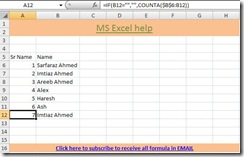## Monday, July 27, 2009

### Sequence formula

Many a times you must want MS Excel to sequence the entry automatically. But thats not one of the features of MS Excel. So what you do is you enter the list than you type 1 in the top most row and drag it till last row. isn't it? This is most common practice I have seen around. Today here I will share to tricks on sequence.

In first sequence type, we will count the based on number of rows filled without checking if the value is duplicate with respect to previous row.

Formula: =IF(B6="","",COUNTA(\$B\$6:B6))

In the above example, we are considering that we are sequencing based on column B starting from 6th row. The formula check if the value is present in B column and then counta find the count of non-blank from column B6 to respective row and returns count. Like, if you are on row 12 then formula will be  =IF(B12="","",COUNTA(\$B\$6:B12)).  Lets take a look at illustration in image below.

Click on image below to view enlargeIn second type, the increment happens only if the text entered is different from the one in the previous cell. Like if John is entered in B2 and B3 then both will have sequence number 1. Remember the same number will be assigned only if the both entered text are in adjacent cells like B2 and B3. Also, if you are using this for data entry purpose than to fix the sequence you can sort on the column.

Click on image below to view enlarge

Formula: =IF(B5="","",IF(B5=B4,A4,A4+1))

First IF checks whether the cells has any value. If the cells is empty then it leaves sequence cell empty(in above example A column has sequence numbers) empty. Else, it checks if the current cells values is same as cell in previous row. if yes, then it returns sequence of previous cell otherwise it adds 1 to sequence of previous row and return new number in sequence. You can concatenate/combine this sequence number with any text to get unique keys like in invoices/bill or memo.

I would suggest you to download the file I have enclosed with post. That will give you more clear picture. Also, I would suggest you to use this trick in one of your MS Excel report or template. So, you can remember entire logic.

Sequence example

Thank you for spending your valuable time in reading above post and if you like to read more such tricks subscribe to blog via email.

We assure you knowledge, not SPAM!